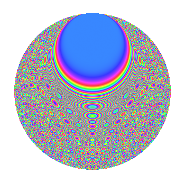# Properties

 Label 525.4.bfLevel $525$ Weight $4$ Character orbit 525.bf Rep. character $\chi_{525}(32,\cdot)$ Character field $\Q(\zeta_{12})$ Dimension $560$ Sturm bound $320$

# Related objects

## Defining parameters

 Level: $$N$$ $$=$$ $$525 = 3 \cdot 5^{2} \cdot 7$$ Weight: $$k$$ $$=$$ $$4$$ Character orbit: $$[\chi]$$ $$=$$ 525.bf (of order $$12$$ and degree $$4$$) Character conductor: $$\operatorname{cond}(\chi)$$ $$=$$ $$105$$ Character field: $$\Q(\zeta_{12})$$ Sturm bound: $$320$$

## Dimensions

The following table gives the dimensions of various subspaces of $$M_{4}(525, [\chi])$$.

Total New Old
Modular forms 1008 592 416
Cusp forms 912 560 352
Eisenstein series 96 32 64

## Trace form

 $$560q + 2q^{3} - 48q^{6} - 4q^{7} + O(q^{10})$$ $$560q + 2q^{3} - 48q^{6} - 4q^{7} + 94q^{12} + 16q^{13} + 4088q^{16} - 14q^{18} + 272q^{21} + 32q^{22} + 32q^{27} - 776q^{28} - 152q^{31} + 430q^{33} + 176q^{36} - 212q^{37} + 910q^{42} + 1360q^{43} - 2024q^{46} - 1604q^{48} + 524q^{51} - 68q^{52} - 3548q^{57} + 1572q^{58} - 968q^{61} + 2974q^{63} + 2764q^{66} + 1012q^{67} - 866q^{72} - 2804q^{73} + 1824q^{76} - 6888q^{78} + 5972q^{81} + 1112q^{82} + 52q^{87} - 1284q^{88} + 7976q^{91} - 3034q^{93} - 492q^{96} - 7496q^{97} + O(q^{100})$$

## Decomposition of $$S_{4}^{\mathrm{new}}(525, [\chi])$$ into newform subspaces

The newforms in this space have not yet been added to the LMFDB.

## Decomposition of $$S_{4}^{\mathrm{old}}(525, [\chi])$$ into lower level spaces

$$S_{4}^{\mathrm{old}}(525, [\chi]) \cong$$ $$S_{4}^{\mathrm{new}}(105, [\chi])$$$$^{\oplus 2}$$

## Hecke characteristic polynomials

There are no characteristic polynomials of Hecke operators in the database